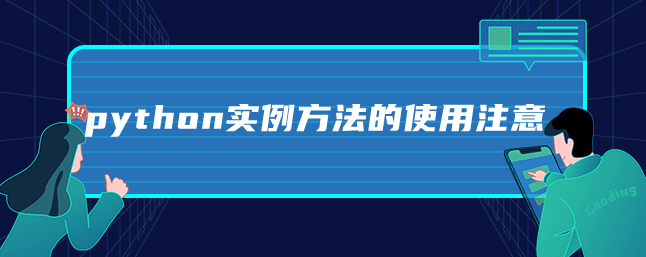# python实例方法的使用注意1、实例方法是从属于实例对象的方法，定义实例方法时，第一个参数必须为 self。self 指当前的实例对象。

2、调用实例方法是，不需要也不能给 self 传值，self 由解释器自动传参。

python实例的使用实例

```class getMin():
# 实例方法
def fun(self, arr, n):
print(arr[n-1])
# 类方法方法
@classmethod
def class_fun(cls):
print("this is class function")

if __name__ == "__main__":
arr = input().strip().split(" ")
int_arr = []
for item in arr:
int_arr.append(int(item))
n = int(input())

instance = getMin()
# 用实例调用实例方法
instance.fun(int_arr, n)
# 用类调用方法
getMin.fun(instance, int_arr, n)
# 实例调用类方法
instance.class_fun()
# 类调用类方法
getMin.class_fun()```

Python开发开发项目